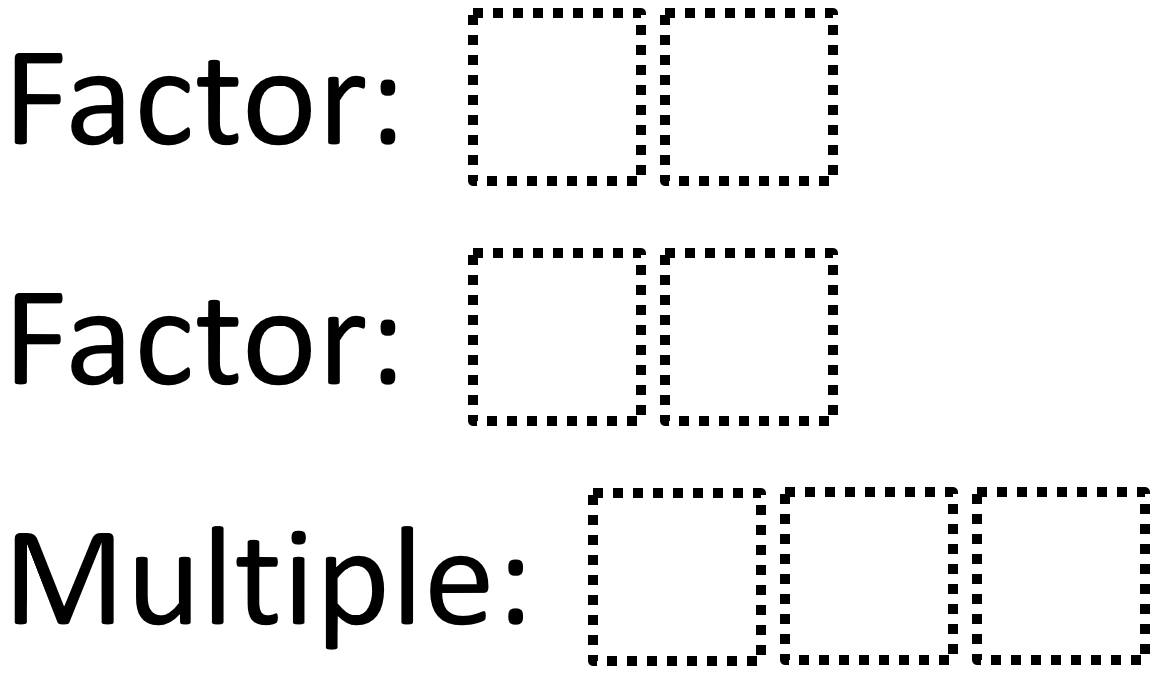# Least Common Multiple

Directions: Use the digits 0 to 9, at most one time each, to fill in the boxes and make a true statement.### Hint

How does choosing the digit for the ones’ place affect the least common multiple?

One answer is LCM 28 and 35 is 140. There may be others.

Source: Wendy Taylor

## Solving One-Step Inequalities with Addition

Directions: Using the digits 0 to 9 at most one time each, place a digit …

1.Another is LCM of 07 and 24 is 168

2.We found 52 x 09 = 468

3.LCM of 02 and 19 is 38

•You need the multiple to be a 3 digit number, so this would not work because you would use zero twice.

4.•same as above

5.LCM of 36 and 45 is 180.

6.I found 01 with 23 which is obviously 23

7.LCM 15×25=375 I dont know right this answer